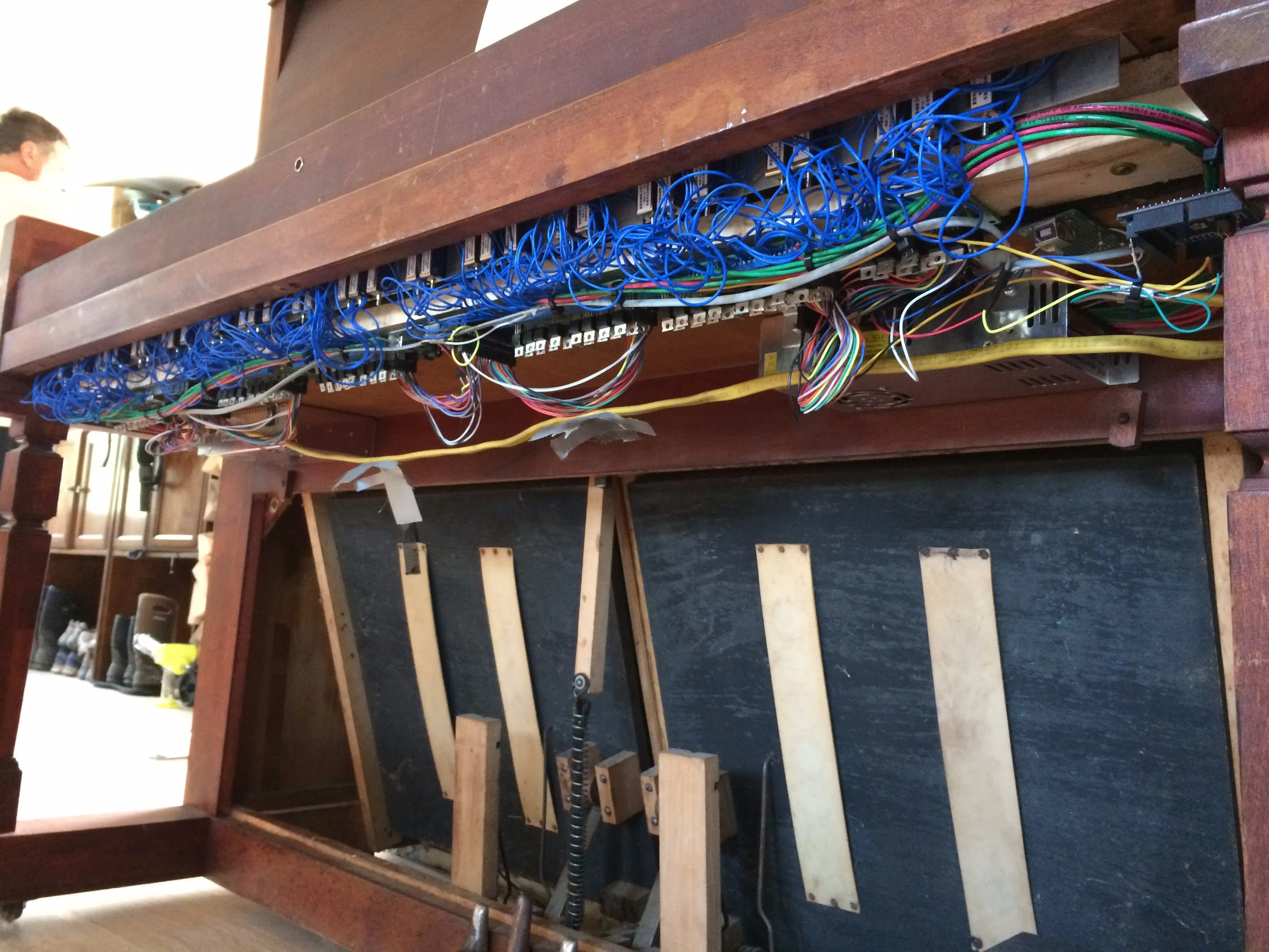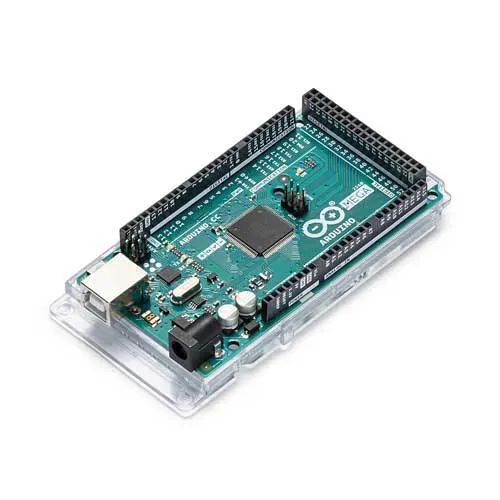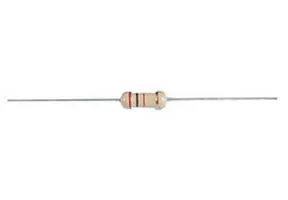Project in progress# Bluetooth MIDI-Operated Antique Reed Organ © GPL3+

Antique reed organ + solenoids + custom driver boards + 3 Arduino Megas + Bluetooth = MIDI-driven reed organ

• 9,120 views
• 28 respects

## Components and suppliesArduino Mega 2560
×3
 ZYE1-0530 Solenoid
×88
 Custom Solenoid Driver Board Design files attached. I manufactured 15@ FirstPCB.com for a total cost of \$50 shipped.
×1
 IRLZ-44N MOSFET
×1Resistor 220 ohm
×1HC-06 Bluetooth Module
×1

## Apps and online services

 Hairless MIDI < - > Serial Bridge This software allows us to send MIDI data from any application on your computer to a serial port, in this case the Bluetooth reciever, which the Arduinos then respond to.

This page was used for a brief description of the project before I published it on Instructables.com. Please view the much more detailed Instructable here: https://www.instructables.com/id/MIDI-Controlled-88-Key-Reed-Organ/

For my high-school senior project, I was going to convert an upright piano to be a MIDI-controlled player piano. However, I by chance ran across a reed organ, and knowing nothing about them became very interested in their sound and history. So I instead converted an Estey Reed organ to be MIDI operated. This is the result.

For now, just the keys are MIDI controlled. I plan on adding a vacuum motor so it doesn't have to be foot pumped, as well as control of the stops on the cheekblocks.

I am currently writing a very detailed Instructable. For now, here are some photos and some demo videos.

Suggest a song or upload a MIDI file for it to play here: http://bit.ly/2wR3QUE

## Code

##### Arduino #1Arduino
For the left-most Arduino
```byte incomingByte;
byte note;
byte velocity;

int statusLed = 13;   // select the pin for the LED

int action = 2; //0 =note off ; 1=note on ; 2= nada

//setup: declaring iputs and outputs and begin serial
void setup() {
pinMode(statusLed, OUTPUT);  // declare the LED's pin as output

int inMin = 22; // Lowest input pin
int inMax = 53; // Highest input pin
for (int i = inMin; i <= inMax; i++) {
pinMode(i, OUTPUT);
}

Serial1.begin(38400);
digitalWrite(statusLed, HIGH);
}

//loop: wait for serial data, and interpret the message
void loop () {
if (Serial1.available() > 0) {

// wait for as status-byte, channel 1, note on or off
if (incomingByte == 144) { // note on message starting starting
action = 1;
} else if (incomingByte == 128) { // note off message starting
action = 0;
} else if (incomingByte == 208) { // aftertouch message starting
//not implemented yet
} else if (incomingByte == 160) { // polypressure message starting
//not implemented yet
} else if ( (action == 0) && (note == 0) ) { // if we received a "note off", we wait for which note (databyte)
note = incomingByte;
playNote(note, 0);
note = 0;
velocity = 0;
action = 2;
} else if ( (action == 1) && (note == 0) ) { // if we received a "note on", we wait for the note (databyte)
note = incomingByte;
} else if ( (action == 1) && (note != 0) ) { // ...and then the velocity
velocity = incomingByte;
playNote(note, velocity);
note = 0;
velocity = 0;
action = 0;
} else {
}
}
}

digitalWrite(statusLed, HIGH);
delay(100);
digitalWrite(statusLed, LOW);
delay(100);
}

void playNote(byte note, byte velocity) {
int value = LOW;
if (velocity > 10) {
value = HIGH;
} else {
value = LOW;
}

if (note > 20 && note < 55) {
digitalWrite((note + 1), value);
}
}
```
##### Arduino #2Arduino
Center Arduino code. All Arduinos are running essentially the same code, they just respond to different MIDI note ranges.
```byte incomingByte;
byte note;
byte velocity;

int statusLed = 13;   // select the pin for the LED

int action = 2; //0 =note off ; 1=note on ; 2= nada

//setup: declaring iputs and outputs and begin serial
void setup() {
pinMode(statusLed, OUTPUT);  // declare the LED's pin as output

int inMin = 22; // Lowest input pin
int inMax = 53; // Highest input pin
for (int i = inMin; i <= inMax; i++) {
pinMode(i, OUTPUT);
}

Serial1.begin(38400);
digitalWrite(statusLed, HIGH);
}

//loop: wait for serial data, and interpret the message
void loop () {
if (Serial1.available() > 0) {

// wait for as status-byte, channel 1, note on or off
if (incomingByte == 144) { // note on message starting starting
action = 1;
} else if (incomingByte == 128) { // note off message starting
action = 0;
} else if (incomingByte == 208) { // aftertouch message starting
//not implemented yet
} else if (incomingByte == 160) { // polypressure message starting
//not implemented yet
} else if ( (action == 0) && (note == 0) ) { // if we received a "note off", we wait for which note (databyte)
note = incomingByte;
playNote(note, 0);
note = 0;
velocity = 0;
action = 2;
} else if ( (action == 1) && (note == 0) ) { // if we received a "note on", we wait for the note (databyte)
note = incomingByte;
} else if ( (action == 1) && (note != 0) ) { // ...and then the velocity
velocity = incomingByte;
playNote(note, velocity);
note = 0;
velocity = 0;
action = 0;
} else {
}
}
}

digitalWrite(statusLed, HIGH);
delay(100);
digitalWrite(statusLed, LOW);
delay(100);
}

void playNote(byte note, byte velocity) {
int value = LOW;
if (velocity > 10) {
value = HIGH;
} else {
value = LOW;
}

if (note > 52 && note < 85) {
digitalWrite((note - 31), value);
}
}
```
##### Arduino #3Arduino
Right Arduino. All Arduinos are running essentially the same code, they just respond to different MIDI note ranges.
```byte incomingByte;
byte note;
byte velocity;

int statusLed = 13;   // select the pin for the LED

int action = 2; //0 =note off ; 1=note on ; 2= nada

//setup: declaring iputs and outputs and begin serial
void setup() {
pinMode(statusLed, OUTPUT);  // declare the LED's pin as output

int inMin = 22; // Lowest input pin
int inMax = 53; // Highest input pin
for (int i = inMin; i <= inMax; i++) {
pinMode(i, OUTPUT);
}

Serial1.begin(38400);
digitalWrite(statusLed, LOW);
}

//loop: wait for serial data, and interpret the message
void loop () {
if (Serial1.available() > 0) {
digitalWrite(statusLed, HIGH);

// wait for as status-byte, channel 1, note on or off
if (incomingByte == 144) { // note on message starting starting
action = 1;
} else if (incomingByte == 128) { // note off message starting
action = 0;
} else if (incomingByte == 208) { // aftertouch message starting
//not implemented yet
} else if (incomingByte == 160) { // polypressure message starting
//not implemented yet
} else if ( (action == 0) && (note == 0) ) { // if we received a "note off", we wait for which note (databyte)
note = incomingByte;
playNote(note, 0);
note = 0;
velocity = 0;
action = 2;
} else if ( (action == 1) && (note == 0) ) { // if we received a "note on", we wait for the note (databyte)
note = incomingByte;
} else if ( (action == 1) && (note != 0) ) { // ...and then the velocity
velocity = incomingByte;
playNote(note, velocity);
note = 0;
velocity = 0;
action = 0;
} else {
}
}
}

digitalWrite(statusLed, HIGH);
delay(100);
digitalWrite(statusLed, LOW);
delay(100);
}

void playNote(byte note, byte velocity) {
int value = LOW;
if (velocity > 10) {
value = HIGH;
} else {
value = LOW;
}

if (note > 84 && note < 109) {
digitalWrite((note - 63), value);
}
}
```

## Schematics

Please note that using this file you can't actually solder in the MOSFETs; the holes are just big enough to jam them in. This makes them easier to replace.
solenoid_driver_board_v4_LbLvMU6CF4.fzz

#### Hammond-Inspired Tonewheel Organ Robot

Project in progress by themixedsignal

• 2,570 views
• 6 respects

#### Arduino Powered Player Pianos and Player Xylophones

Project tutorial by Netcamprojects

• 10,282 views
• 23 respects

#### Bluetooth Controlled & Obstacle Avoiding Arduino Robot

Project showcase by KureBas Robotics

• 27,719 views
• 41 respects

#### Connecting "stuff" via Bluetooth / Android / Arduino

Project tutorial by MJRoBot (Marcelo Rovai)

• 24,498 views
• 52 respects

#### Cthulhinho: A MIDI Sequencer

Project showcase by primvla

• 20,620 views